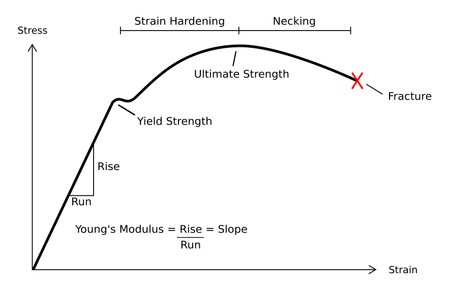# Resilience (materials science)

For other uses, see Resilience (disambiguation).The area under the linear portion of a stress-strain curve is the resilience of the material

In material science, resilience is the ability of a material to absorb energy when it is deformed elastically, and release that energy upon unloading. Proof resilience is defined as the maximum energy that can be absorbed up to the elastic limit, without creating a permanent distortion. The modulus of resilience is defined as the maximum energy that can be absorbed per unit volume without creating a permanent distortion. It can be calculated by integrating the stress-strain curve from zero to the elastic limit. In uniaxial tension,where Ur is the modulus of resilience, σy is the yield strength, εy is the yield strain, and E is the Young's modulus.

## Unit of resilience

Resilience (Ur) is measured in a unit of joule per cubic meter (J·m−3) in the SI system, i.e. elastical deformation energy per surface of test specimen (merely for gage-length part).

Like the unit of tensile toughness (UT), the unit of resilience can be easily calculated by using area underneath the stress–strain (σε) curve, which gives resilience value, as given below:

Ur = Area underneath the stress–strain (σε) curve = 1/2' 'xσ x ε

Ur = MPa × % = (N·m−2·106)·(m·m−1·10−2)

Ur = N·m·m−3·104

Ur = J·m−3·104

1. Campbell, Flake C. (2008). Elements of Metallurgy and Engineering Alloys. ASM International. p. 206. ISBN 9780871708670.
2. O.Balkan and H.Demirer (2010). "Polym. Compos.". 31: 1285. ISSN 1548-0569.